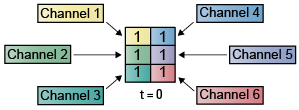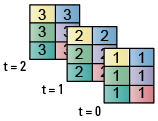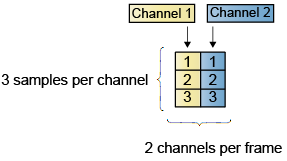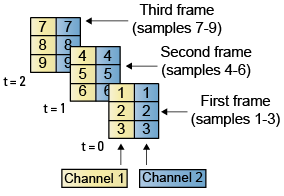## Sample- and Frame-Based Concepts

### Sample- and Frame-Based Signals

Sample-based signals are the most basic type of signal and are the easiest to construct from a real-world (physical) signal. You can create a sample-based signal by sampling a physical signal at a given sample rate, and outputting each individual sample as it is received. In general, most Digital-to-Analog converters output sample-based signals.

You can create frame-based signals from sample-based signals. When you buffer a batch of N samples, you create a frame of data. You can then output sequential frames of data at a rate that is 1/N times the sample rate of the original sample-based signal. The rate at which you output the frames of data is also known as the frame rate of the signal.

Frame-based data is a common format in real-time systems. Data acquisition hardware often operates by accumulating a large number of signal samples at a high rate. The hardware then propagates those samples to the real-time system as a block of data. Doing so maximizes the efficiency of the system by distributing the fixed process overhead across many samples. The faster data acquisition is suspended by slower interrupt processes after each frame is acquired, rather than after each individual sample. See Benefits of Frame-Based Processing for more information.

### Model Sample- and Frame-Based Signals in MATLAB and Simulink

When you process signals using DSP System Toolbox software, you can do so in either a sample- or frame-based manner. When you are working with blocks in Simulink®, you can specify, on a block-by-block basis, which type of processing the block performs. In most cases, you specify the processing mode by setting the Input processing parameter. When you are using System objects in MATLAB®, only frame-based processing is available. The following table shows the common parameter settings you can use to perform sample- and frame-based processing in MATLAB and Simulink.

Sample-Based ProcessingFrame-Based Processing
Simulink — BlocksInput processing = ```Elements as channels (sample based)```Input processing = ```Columns as channels (frame based)```

### What Is Sample-Based Processing?

In sample-based processing, blocks process signals one sample at a time. Each element of the input signal represents one sample in a distinct channel. For example, from a sample-based processing perspective, the following 3-by-2 matrix contains the first sample in each of six independent channels.When you configure a block to perform sample-based processing, the block interprets scalar input as a single-channel signal. Similarly, the block interprets an M-by-N matrix as multichannel signal with M*N independent channels. For example, in sample-based processing, blocks interpret the following sequence of 3-by-2 matrices as a six-channel signal.For more information about the recent changes to frame-based processing, see the Frame-based processing changes section of the DSP System Toolbox Release Notes.

### What Is Frame-Based Processing?

In frame-based processing, blocks process data one frame at a time. Each frame of data contains sequential samples from an independent channel. Each channel is represented by a column of the input signal. For example, from a frame-based processing perspective, the following 3-by-2 matrix has two channels, each of which contains three samples.When you configure a block to perform frame-based processing, the block interprets an M-by-1 vector as a single-channel signal containing M samples per frame. Similarly, the block interprets an M-by-N matrix as a multichannel signal with N independent channels and M samples per channel. For example, in frame-based processing, blocks interpret the following sequence of 3-by-2 matrices as a two-channel signal with a frame size of 3.Using frame-based processing is advantageous for many signal processing applications because you can process multiple samples at once. By buffering your data into frames and processing multisample frames of data, you can often improve the computational time of your signal processing algorithms. To perform frame-based processing, you must have a DSP System Toolbox license.

For more information about the recent changes to frame-based processing, see the Frame-based processing changes section of the DSP System Toolbox Release Notes.

#### Benefits of Frame-Based Processing

Frame-based processing is an established method of accelerating both real-time systems and model simulations.

Accelerate Real-Time Systems.  Frame-based data is a common format in real-time systems. Data acquisition hardware often operates by accumulating a large number of signal samples at a high rate, and then propagating those samples to the real-time system as a block of data. This type of propagation maximizes the efficiency of the system by distributing the fixed process overhead across many samples; the faster data acquisition is suspended by slower interrupt processes after each frame is acquired, rather than after each individual sample is acquired.

The following figure illustrates how frame-based processing increases throughput. The thin blocks each represent the time elapsed during acquisition of a sample. The thicker blocks each represent the time elapsed during the interrupt service routine (ISR) that reads the data from the hardware.

In this example, the frame-based operation acquires a frame of 16 samples between each ISR. Thus, the frame-based throughput rate is many times higher than the sample-based alternative.Be aware that frame-based processing introduces a certain amount of latency into a process due to the inherent lag in buffering the initial frame. In many instances, however, you can select frame sizes that improve throughput without creating unacceptable latencies. For more information, see Delay and Latency.

Accelerate Model Simulations.  The simulation of your model also benefits from frame-based processing. In this case, you reduce the overhead of block-to-block communications by propagating frames of data rather than individual samples.

## Related Examples